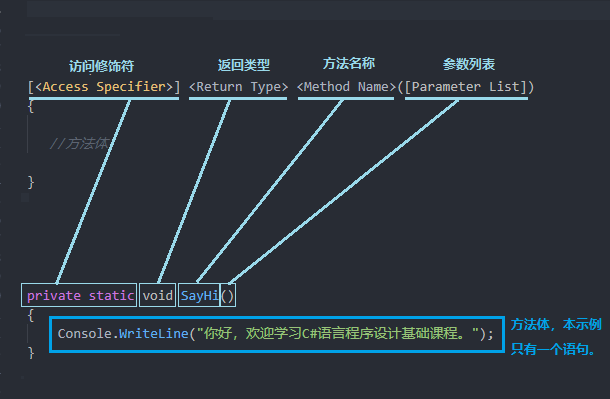# C#方法(Method)

1890 更新于: 2021-07-17 读完约需 5 分钟

## 概述

• 如何定义一个C#的方法(了解方法的语法结构)
• 如何调用方法

## 方法的语法结构

``````[<Access Specifier>] <Return Type> <Method Name>([Parameter List])
{

//方法体

}
``````

``````private static void SayHi()
{
Console.WriteLine("你好，欢迎学习C#语言程序设计基础课程。");
}
``````• 访问修饰符(可选)：是C#语言中所有类型或者类型成员拥有的可以被访问的级别，将在后续的章节中具体讲解。

• 返回类型: 返回类型是方法返回的值的数据类型，可以是C#支持的任意数据类型。如果该方法不返回任何值，则返回类型为`void`

• 方法名称: 方法名称可以重复(并且区分大小写)，但同一个类或者结构中的方法签名不允许重复，方法签名是唯一的标识符。它不能与类中声明的任何其他标识符相同，命名方法名称时应尽量有意义，命名规则使用PascalCase(帕斯卡)命名法

• 参数列表: 调用方法时需要传入的参数，位于方法名称后的一对中括号内。参数列表指的是方法参数的类型、顺序和数量。参数是可选的;也就是说，一个方法可能不包含参数，参数名使用camelCase命名法

• 方法体: 它包含完成所需活动所需的指令集(代码块)

## 方法的定义和调用

``````static void Main()
{
SayHi();
}
``````

## 方法的实际使用案例

### 不使用方法封装实现两个数比较大小

``````int x,y;
x = 2;
y = 3;
int max;
if (x >= y)
{
max = x;
}
else
{
max = y;
}

Console.WriteLine("x={0},y={1},较大数为:{2}",x,y,max);

x = 5;
y = 1;
max = 0;
if (x >= y)
{
max = x;
}
else
{
max = y;
}

Console.WriteLine("x={0},y={1},较大数为:{2}", x, y, max);
``````

### 使用方法封装实现两个数比较大小

``````static void Main(string[] args)
{
int x, y;
x = 2;
y = 3;
int max = FindMax(x,y);
Console.WriteLine("x={0},y={1},较大数为:{2}", x, y, max);

x = 50;
y = 20;
max = FindMax(x,y);
Console.WriteLine("x={0},y={1},较大数为:{2}", x, y, max);

// 或者不使用中间变量，直接调用方法
x = 2;
y = 3;
Console.WriteLine("x={0},y={1},较大数为:{2}", x, y, FindMax(x, y));

x = 50;
y = 20;
Console.WriteLine("x={0},y={1},较大数为:{2}", x, y, FindMax(x, y));

}

static int FindMax(int x,int y)
{
int max;
if (x >= y)
{
max = x;
}
else
{
max = y;
}
return max;
}
``````

``````private static void SayHi(string customerName)
{
Console.WriteLine("你好，{0}",customerName);
}
``````

``````private static int Sum(int x,int y)
{
int result = x + y;
return result;
}
``````

## 直接调用方法

``````Console.WriteLine(Sum(2,3));
``````

## 将一个方法的返回值赋给变量

``````int sum = Sum(2,3);
Console.WriteLine(sum);
``````

## 方法的参数可以是表达式

``````int sum = Sum((2*3),3);
Console.WriteLine(sum);
``````

## 方法的重载

``````static double FindMax(double x,double y)
{
double max;
if (x >= y)
{
max = x;
}
else
{
max = y;
}
return max;
}
``````

## 可选参数和命名参数

``````/// <summary>
/// 打招呼的方法(命名参数)
/// </summary>
/// <param name="greeting"></param>
static void SayHiNamed(string username, string greeting)
{
}

/// <summary>
/// 打招呼的方法(可选参数及参数默认值)
/// </summary>
/// <param name="greeting"></param>
static void SayHiOptional(string username, string greeting = "Hi")
{
}

/// <summary>
/// 打招呼的方法(可选参数)
/// </summary>
/// <param name="greeting"></param>
static void SayHi(string username = "Unknown", string greeting = "Hi",bool showGreeting = true)
{
if (!showGreeting)
{
}
else
{
}
}
``````

``````Console.WriteLine("*********命名参数**********");
SayHiNamed("Rector", "Hi");

Console.WriteLine("**********可选参数**********");
SayHiOptional("Rector");
SayHiOptional("Rector", "你好");

Console.WriteLine("**********默认参数值**********");
SayHi();
SayHi("Rector", "Hello");
SayHi(greeting: "Hello");
``````

``````    **********命名参数**********
Hi, Rector
Hello, Rector
**********可选参数**********
Hi, Rector
你好, Rector
Hello, Rector
**********默认参数值**********
Hi, Unknown
Hi, Rector
Hello, Rector
Hello, Unknown
Hello, Liu
Liu
``````